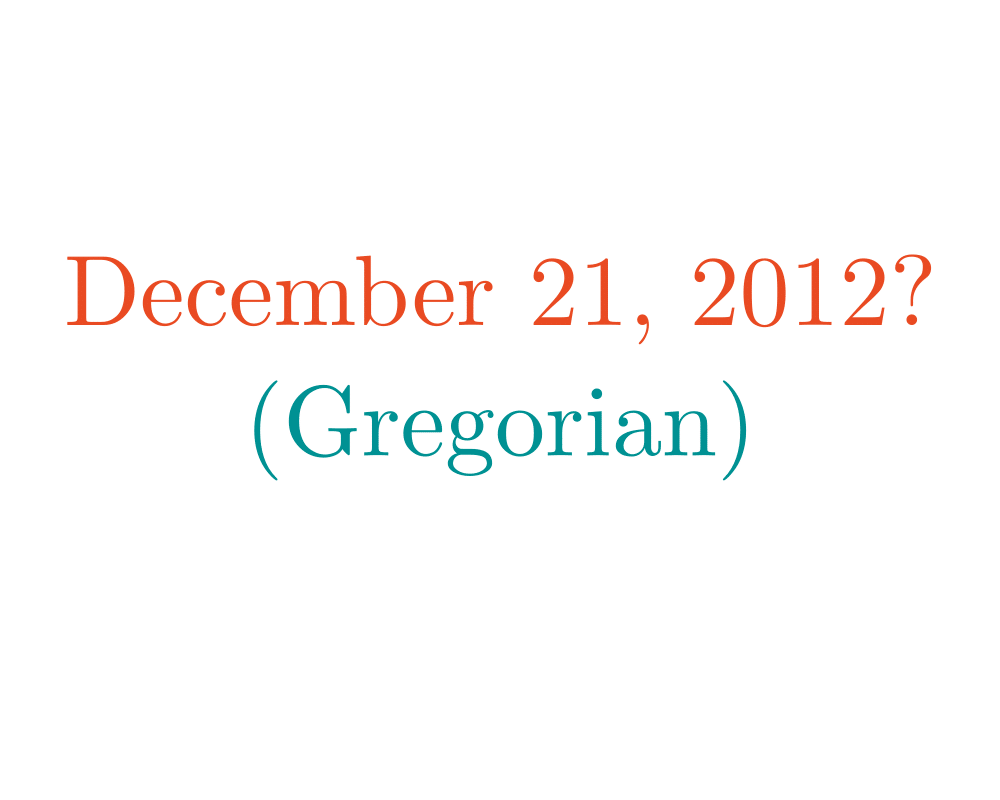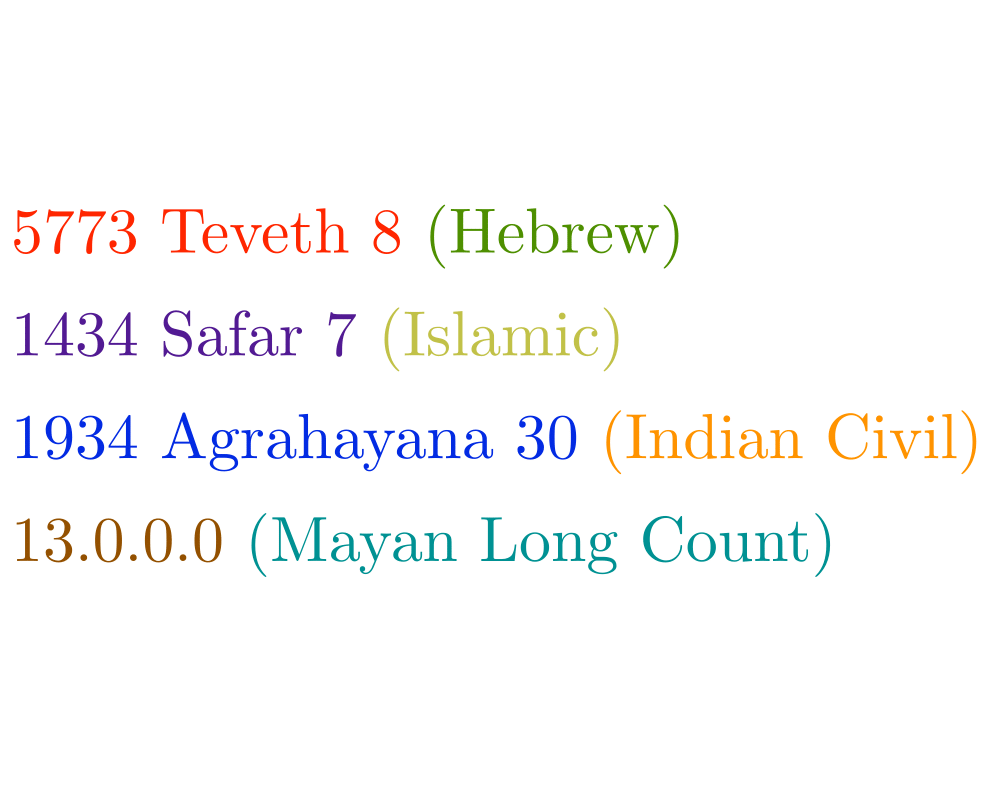# Calendrical CalculationsInputOutput

Input Description: A particular calendar date $$d$$, specified by month, day and year.
Problem: Which day of the week did $$d$$ fall on in the given calendar system?

Excerpt from The Algorithm Design Manual: Many business applications need to perform calendrical calculations. Perhaps we want to display a calendar of a specified month and year. Maybe we need to compute what day of the week or year some event occurs, as in figuring out the date on which a 180-day futures contract comes due. The importance of correct calendrical calculations is perhaps best revealed by the furor over the millennium bug,'' the crisis in legacy programs that allocate only two digits for storing the year.

More complicated questions arise in international applications, because different nations and ethnic groups around the world use different calendar systems. Some of these, like the Gregorian calendar used in most of the world, are based on the sun, while others, like the Hebrew calendar, are lunar calendars. How would you tell today's date according to the Chinese or Arabic calendar?

The algorithms associated with calendrical calculations are different from the other problems in this book, because calendars are historical objects, not mathematical ones. The issues revolve around specifying the rules of the calendrical system and simply implementing them correctly, rather than designing efficient shortcuts for the computation.

The basic approach behind calendar systems is to start with a particular reference date and count from there. The particular rules for wrapping the count around into months and years is what distinguishes a given calendar system from another. To implement a calendar, we need two functions, one that given a date returns the integer number of days that have elapsed since the reference start date, the other of which takes an integer $$n$$ and returns the calendar date exactly $$n$$ days from the reference date. This is analogous to the ranking and unranking rules for combinatorial objects, such as permutations.

Go To Main Page